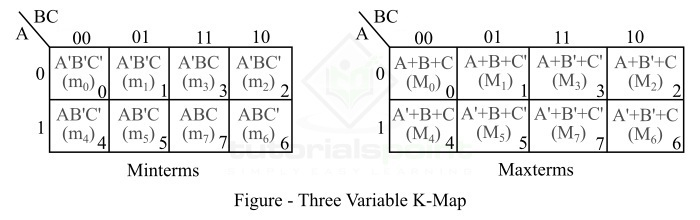# 3 Variable K-Map in Digital Electronics

A K-Map or Karnaugh Map is a graphical method that used for simplifying the complex algebraic expressions in Boolean functions. This method avoid the use of complex theorems and equations manipulations. A K-Map is basically a special form of a truth table that can easily map out the values of parameters and gives a simplified Boolean expression.

K-Map method is best suited for such Boolean functions that have two to four variables. However, it can be used for Boolean functions having five or six variables as well, but its process becomes more difficult with the increased number of variables in the function.

Therefore, in practice, we mostly use Two-Variable K-Map, Three-Variable K-Map, and Four-Variable K-Map. But, sometimes, the Five-Variable K-Map and Six-Variable K-Map are also used to derive the Boolean expressions.

In this tutorial, we will discuss the 3 Variable K-Map and its application to simplify a complex Boolean function.

## Three-Variable K-Map

We can use the K-Map to simplify a Boolean function of three-variables. A Boolean function in three variables (A, B, C) can be expressed in the standard sum of product (SOP) form that can have total eight possible combinations, which are as follows −

$$\mathrm{(A'B'C'), (A'B'C), (A'BC'), (A'BC), (AB'C'), (AB'C), (ABC'), (ABC)}$$

We can designate each of these combinations by m0, m1, m2, m3, m4, m5, m6, and m7 respectively. Each of these terms are called a min-term. In these combinations, A is called MSB (Most Significant Bit) and C is called LSB (Least Significant Bit).

In terms of POS (Product of Sum) form, the eight possible combinations of the three variables Boolean expression are as follows −

$$\mathrm{(A+B+C), (A+B+C'), (A+B'+C), (A+B'+C'), (A'+B+C), (A'+B+C'), (A'+B'+C), (A'+B'+C')}$$

Each one of these combinations are often designated by M0, M1, M2, M3, M4, M5, M6, and M7 respectively. Each of these terms is called a maxterm. Similar to the minterm, A is called MSB (Most Significant Bit) and C is called LSB (Least Significant Bit).

Therefore, a three variable K-Map has eight (23) squares or cells, and each square on the K-Map represents a minterm of a maxterm as shown in the following figure.Here, the small number on the bottom right corner of each cell indicates the minterm or maxterm designation.

The binary numbers along the top of the K-Map indicates the condition of variables B and C for each column. The binary number along the left side of the map against each row represents the condition of the variable A for that row.

For example, the binary number of 10 on the top of the fourth column in the above figure indicates that the variable B appears in non-complimented form and the variable C appears in complimented form in all the minterms in that column. The binary number 0 on the left of the first row on the K-map indicates that the variable A appears in its complimented form in all the minterms, and the binary number 1 on the left of the second row on the K-Map indicates that the variable A appears in its non-complimented form in all the minterms.

Also, note that the binary numbers on top of the K-map are not in the normal binary order, but they are actually in the Gray code. The use of Gray code in K-map ensures that the two physically adjacent cells are actually adjacent which means their minterms or maxterms differs by one variable only.

## Numerical Example

Map the following three-variable Boolean expression on K-Map.

$$\mathrm{f=\overline{A}\, \overline{B}\,C+A\,\overline{B}\,C+\overline{A}\,B\,\overline{C}+A\,\overline{B}\,\overline{C}+ABC}$$

### Solution

In the given Boolean expression, the minterms are −

$$\mathrm{\overline{A}\,\overline{B}\,C=001;\:A\,\overline{B}\,C=101;\: \overline{A}\,B\,\overline{C}=010;\:A\,\overline{B}\,\overline{C}=100;\: ABC=111}$$

Therefore,

$$\mathrm{m_{1}=\overline{A}\,\overline{B}\,C=001}$$

$$\mathrm{m_{5}=A\,\overline{B}\,C=101}$$

$$\mathrm{m_{2}=\overline{A}\,B\,\overline{C}=010}$$

$$\mathrm{m_{4}=A\,\overline{B}\,\overline{C}=100}$$

$$\mathrm{m_{7}=ABC=111}$$

Hence, the expression is given by,

$$f=\sum m\left ( 1,5,2,4,7 \right )$$

The K-map of this expression is shown in the following figure −## Conclusion

From the above discussion, we may conclude that the three variable K-Map is a graphical method used to simplify the complex three variable Boolean function. A three-variable Kmap has eight squares or cells.

Updated on: 26-Dec-2022

3K+ Views